﻿ Material with Temperature-Dependent Anisotropic ElasticityExamples | Product | Murata Software Co., Ltd.Example31Material with Temperature-Dependent Anisotropic Elasticity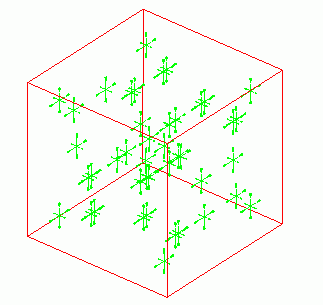General

• The material has the temperature-dependent anisotropic elasticity. The deformation under thermal load is analyzed.

• Multi-step thermal load analysis is performed. There are multiple reached temperatures. The stresses for each temperature are solved.

• Unless specified in the list below, the default conditions will be applied.

Analysis Space

 Item Settings Analysis Space 3D Model unit mm

Analysis Conditions

The temperature is applied evenly on the model.

Opt for the thermal load in the analysis condition, and set the reference temperature and the reached temperature.

There is no need to couple with the thermal analysis [Watt].

 Item Settings Solvers Mechanical Stress Analysis [Galileo] Analysis Type Static analysis Options Select “Thermal load”.

The Step/Thermal Load tab is set as follows.

In this setting, thermal load analysis is performed with 3 reached temperatures step by step.

Tab

Setting Item

Settings

Step Setting

Reference temperature

25[deg]

Step/Reached Temperature Setting

 Step Substeps Reached temperature [deg] 1 1 50 2 1 75 3 1 100

Model

The model is a cubic solid body. The material has the temperature-dependent anisotropic coefficient of expansion. The outer boundary condition is

set with no displacement.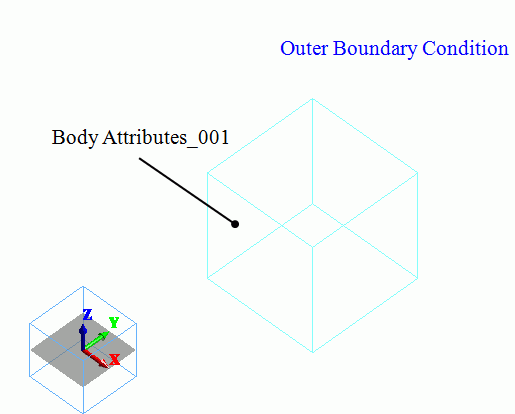Body Attributes and Materials

The material properties are set up as follows: The temperature dependency for the elasticity is set for 50, 75, and 100 [deg].

 Material Name Tab Properties Material_Property_001 Elasticity Material Type: Elastic/Anisotropic Temperature Dependency: Yes   The temperature dependency of the elasticity is set as follows. Subscripts 1, 2 and 3 indicate x, y and z components respectively.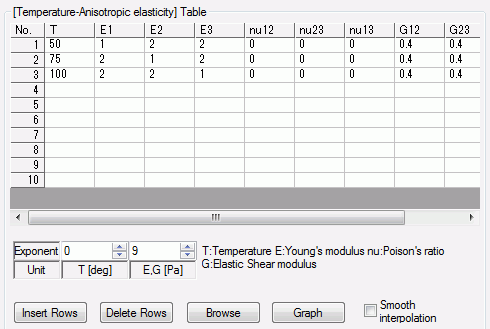* This is not the actual material’s property. Coefficient of Expansion Temperature Dependency: No Anisotropy: Isotropic Coefficient of Expansion : 10×10^-6[1/deg]

Boundary Conditions

 Boundary Condition Name/Topology Tab Boundary Condition Type Settings Outer Boundary Condition Mechanical Displacement Select all X/Y/Z components. UX=0, UY=0, UZ=0

Results

The figure below shows the principal stress at 50 deg.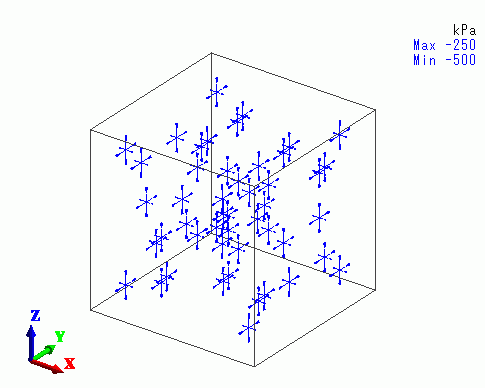At 50 deg, the compressive stress is smaller in X direction, as the X component of Young’s modulus is smaller than the others.

The figure below shows the principal stress at 75 deg.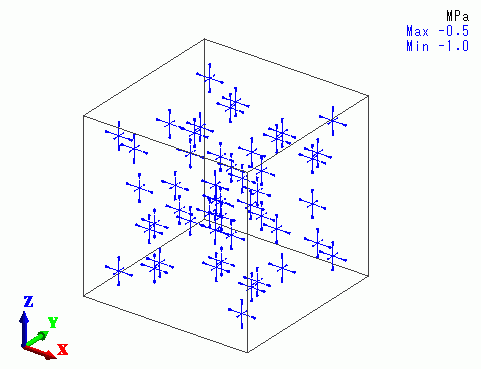At 75 deg, the compressive stress is smaller in Y direction, as the Y component of Young’s modulus is smaller than the others.

The figure below shows the principal stress at 100 deg.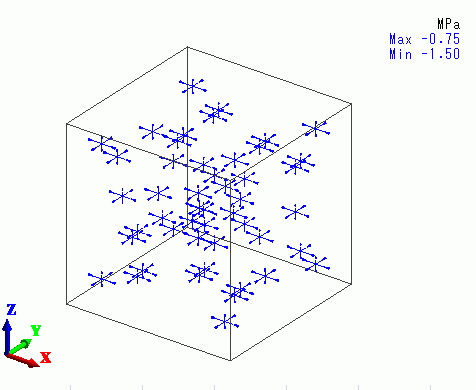At 100 deg, the compressive stress is smaller in Z direction, as the Z component of Young’s modulus is smaller than the others.

﻿